# Capacitors Types | Testing

Capacitors of different kinds are found nearly in every electronic circuit. A capacitor is basically mean to store electrons (electrical energy) and release them whenever desired. A capacitor consists of two conductors separated by an insulator. The conductors are known as plates and the insulator is known as a dielectric.Capacitance is the measure of capacitor’s ability to store charge. It is measured in farads (F) and represented by C. Because the farad is a large unit, microfarads (10-6 µF) and pico-farads (10-12pF) are used generally.

The capacitance of a capacitor is directly proportional to the area of plates and inversely proportional to the distance between the plates. It also depends upon the dielectric constant of the material used between the plates.

A dielectric constant is a measure of the effectiveness of a material as an insulator. This constant compares the material’s ability to store energy in an electric field to the ability of air, which has a dielectric constant of 1. Paper has a dielectric constant of 2 to 3, mica has a dielectric constant of 5 to 6 and titanium has a dielectric constant of 90 to 170.

A capacitor offers low impedance to AC and high impedance to DC. So capacitors are used where we want to couple alternating voltages from one circuit to another, while at the same time blocking the DC voltage from reaching the next circuit. It is also used as a bypass capacitor, where it bypasses the AC through it.

## Capacitors in Series

Series combination of capacitors decreases the total capacitance of combination. The total capacitance of capacitors in series is calculated like the total resistance of parallel resistors:

1/CT = 1/C1 +1/C2 +1/C3 + ……

## Capacitors in Parallel

Placing capacitors in parallel makes the total capacitance equal to the sum of the individual capacitances:

CT = C1 + C2 + C3 + ……## Capacitor Types

There are many types of capacitors. Following are the most common capacitor types:

Electrolytic Capacitors

Electrolytic capacitors consist of two metal foils separated by fine gauze or other absorbent material that is saturated with a chemical paste called an electrolyte.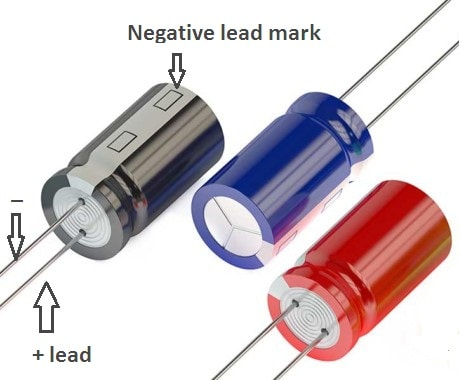The value of electrolytic capacitor may range from 1 µF to several thousand microfarads. The voltage rating may range from 1 V to 500 V or more. These capacitors are used in situations where the large capacitance is required. It has high capacitance to size ratio.

In DC circuits, polarized electrolytic capacitors are used i.e. they have positive and negative leads. Polarity must be observed when connecting an electrolytic capacitor to a DC circuits. Whereas electrolytic capacitors used in AC circuits (for motor starters) have no polarity.

Paper Capacitors

Paper capacitors are constructed by a rolled foil technique. The capacitor consists of two metal rolled foils separated by strips of paper. This paper is impregnated with a dielectric material such as oil, wax or plastic.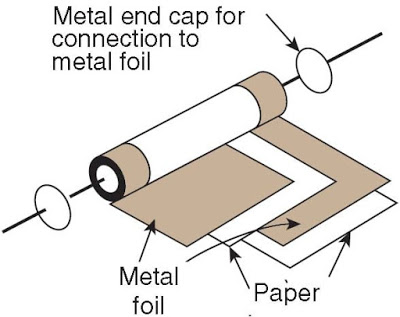Paper capacitors have capacitances ranging from 0.0005 µF to several µF and are rated from about 100V to several thousand volts. They have no polarity. They can be used for both DC and AC.

Ceramic Capacitors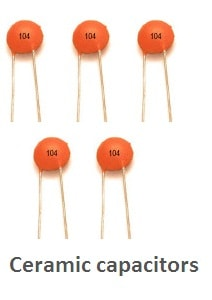Ceramic capacitors are made in many shapes and sizes. The ceramic disk capacitor is popular because it is inexpensive to produce. The ceramic material is the dielectric. This is a tough, reliable, general-purpose capacitor. It is used for capacitors of 0.1 microfarads and smaller. Their working voltage ranges from 3 V to 6000 V. They can be used in both DC and AC circuits.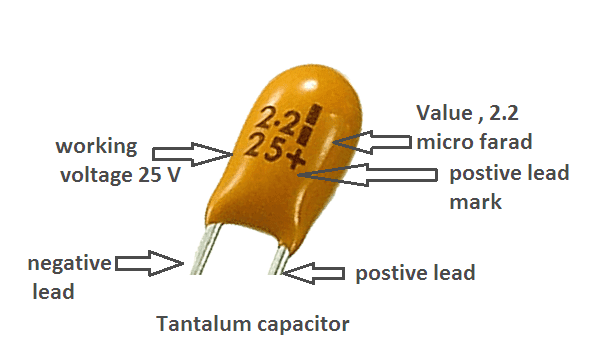Variable Capacitors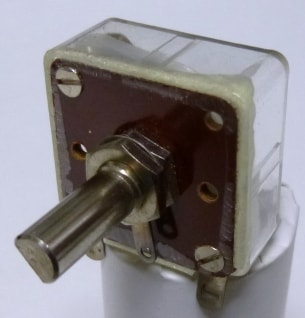In some applications, it is desirable to be able to change the value of capacitance readily. This is done by means of a variable capacitor. The most common variable capacitor is the air-gang capacitor. The dielectric of this capacitor is air. By rotating the shaft at one end, we can change the capacitance of the air-gang capacitor by changing the common area between plates.

In some circuits, the need for variation in capacitance is not frequent. In such situations, we use a variable capacitor known as trimmer or padder. Both ceramic and mica are used as the dielectric for trimmers.

## Color Coding for Capacitors

In the case of large capacitors, its rating is printed on its body. Whereas in the case of small capacitors; its rating is printed on its body, using the color code. The color code is similar to that used for resistances.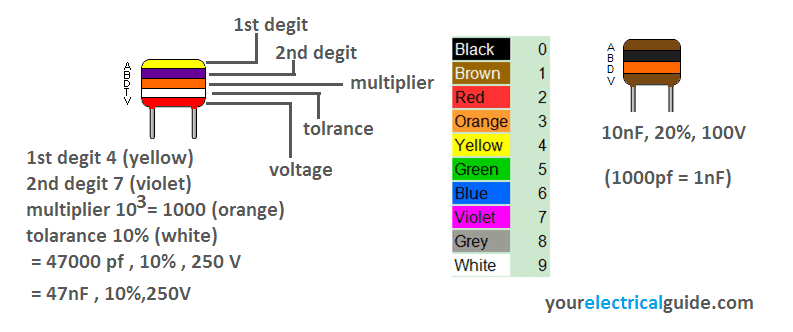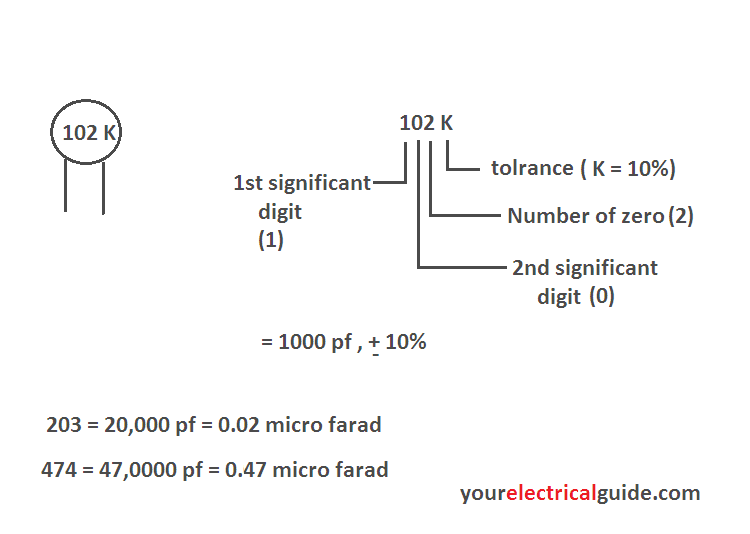## How to test a capacitor?

When a DC voltage source is connected to a capacitor, a current flows until the capacitor is charged. Once the capacitor is charged, current flow stops and the capacitor’s  voltage becomes equal to the voltage of the voltage source. In a DC circuit, a capacitor acts as an open circuit after its initial charge. On the basis of this information, we can test the capacitors easily.

Method 1

This method is used for polarized electrolytic capacitors like 10 MFD 50 V, 1000 MFD 25 V etc. which are normally used as coupling or filter capacitors in electronic circuits.

• Take an analog multi-meter.
• Set the meter on mega ohm range for resistance measurement.
• Measure the resistance of the capacitor.

If a capacitor is good then initially it shows low resistance and after few seconds its resistance gradually increases towards infinite. However, this time of resistance building depends upon the rating of capacitor (capacitance and voltage).

Method 2
This method is used for non polarized capacitors like 2.5 MFD 230 V, 100 MFD 250 V which are normally used for fans, motor starters etc.

• Make sure capacitor is fully discharged (by short circuiting its terminals).
• Connect it to 230 V AC supply for few seconds safely.
• Remove it from supply mains.
• Short circuit the capacitor terminals.

If a strong spark appears, it means capacitor is good otherwise it is weak. However, strength of spark depends upon the rating of capacitor and supply voltage. A charged capacitor should be handled carefully otherwise it may cause a serious electric shock.

Electronics | All Posts